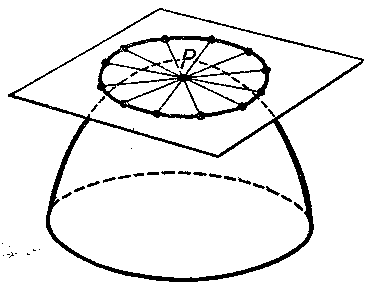# Dupin indicatrix

curvature indicatrix

A plane curve illustrating the normal curvatures of a surface at a point of this surface. The Dupin indicatrix lies in the tangent plane to the surface $S$ at the point $P$ and there it is described by the radius vector $r$ of length $1/\sqrt{|K_r|}$, where $K_r$ is the normal curvature of $S$ at $P$ in the direction $r$. Let $\mathbf r=\mathbf r(u,v)$ be a parametrization of $S$ in a neighbourhood of $P$. One introduces a coordinate system on the tangent plane to $S$ at $P$, taking $P$ as the coordinate origin, and the vectors $\mathbf r_u$ and $\mathbf r_v$ as the basis vectors of this coordinate system. The equation of the Dupin indicatrix will then be

$$|Lx^2+2Mxy+Ny^2|=1,$$

where $x$ and $y$ are the coordinates of a point on the Dupin indicatrix, and $L$, $M$ and $N$ are the coefficients of the second fundamental form of $S$ calculated at $P$. The Dupin indicatrix is: a) an ellipse if $P$ is an elliptic point (a circle if $P$ is an umbilical point); b) a pair of conjugate hyperbolas if $P$ is a hyperbolic point; and c) a pair of parallel straight lines if $P$ is a parabolic point. The curve is named after Ch. Dupin (1813), who was the first to use this curve in the study of surfaces.Figure: d034180a

The Dupin indicatrix at $P$ can be obtained as the limit of suitably normalized (inter)sections of the surface with planes parallel to the tangent plane of $S$ at $P$ which are approaching this plane, see [a1], p. 370; [a2], p. 363-365.